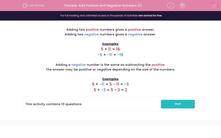# Add Positive and Negative Numbers (1)

In this worksheet, students add together positive and negative numbers.This content is premium and exclusive to EdPlace subscribers.Key stage:  KS 2

Curriculum topic:   Maths and Numerical Reasoning

Curriculum subtopic:   Mixed Problems

Difficulty level:#### Worksheet Overview

Examples

5 + 11 = 16

-5 + -11 = -16

Adding a negative number is the same as subtracting the positive.

The answer may be positive or negative depending on the size of the numbers.

Examples

5 + -11 = 5 - 11 = -6

5 + -3 = 5 - 3 = 2

### What is EdPlace?

We're your National Curriculum aligned online education content provider helping each child succeed in English, maths and science from year 1 to GCSE. With an EdPlace account you’ll be able to track and measure progress, helping each child achieve their best. We build confidence and attainment by personalising each child’s learning at a level that suits them.

Get started••••